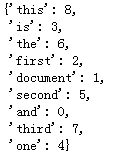#### one-hot

One-hot表示方法的例子如下：

{
‘我’: 1, ‘爱’: 2, ‘北’: 3, ‘京’: 4, ‘天’: 5,
‘安’: 6, ‘门’: 7, ‘喜’: 8, ‘欢’: 9, ‘上’: 10, ‘海’: 11
}

Bag of Words
Bag of Words（词袋表示），也称为Count Vectors，每个文档的字/词可以使用其出现次数来进行表示。

from sklearn.feature_extraction.text import CountVectorizer
corpus = [
'This is the first document.',
'This document is the second document.',
'And this is the third one.',
'Is this the first document?',
]
vectorizer = CountVectorizer()
vectorizer.fit_transform(corpus).toarray()

vectorizer.vocabulary_#### N-gram

N-gram与Count Vectors类似，不过加入了相邻单词组合成为新的单词，并进行计数。

#### TF-IDF

TF-IDF 分数由两部分组成：第一部分是词语频率（Term Frequency），第二部分是逆文档频率（Inverse Document Frequency）。其中计算语料库中文档总数除以含有该词语的文档数量，然后再取对数就是逆文档频率。

TF(t)= 该词语在当前文档出现的次数 / 当前文档中词语的总数
IDF(t)= log_e（文档总数 / 出现该词语的文档总数）

Count Vectors + RidgeClassifier

import pandas as pd

from sklearn.feature_extraction.text import CountVectorizer
from sklearn.linear_model import RidgeClassifier
from sklearn.metrics import f1_score

vectorizer = CountVectorizer(max_features=3000)
train_test = vectorizer.fit_transform(train_df['text'])

clf = RidgeClassifier()
clf.fit(train_test[:10000], train_df['label'].values[:10000])

val_pred = clf.predict(train_test[10000:])
print(f1_score(train_df['label'].values[10000:], val_pred, average='macro'))
#0.74


TF-IDF + RidgeClassifier

import pandas as pd

from sklearn.feature_extraction.text import TfidfVectorizer
from sklearn.linear_model import RidgeClassifier
from sklearn.metrics import f1_score

tfidf = TfidfVectorizer(ngram_range=(1,3), max_features=3000)
train_test = tfidf.fit_transform(train_df['text'])

clf = RidgeClassifier()
clf.fit(train_test[:10000], train_df['label'].values[:10000])

val_pred = clf.predict(train_test[10000:])
print(f1_score(train_df['label'].values[10000:], val_pred, average='macro'))
#0.87


07-2636
07-253807-2569
08-0366
07-2536
07-2547
07-2542
07-2750